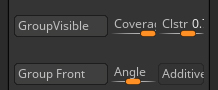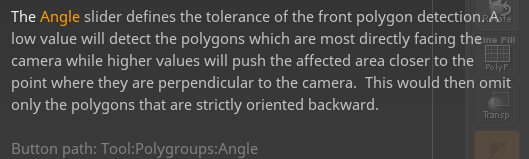# Can blender do this?

Hi all,
I m looking for a way to make a shader that blends 2 colors based on an angle offset from the global x direction.
Im uploading an image but could make the mix be controlled by angle.
This is similar to zbrush group front with an angle slider.

This feature is useful in 3dprinting for parting.

Thanks in advance!what is angle offset?

heres an explanation for the angle
thxTake a dot product between world space normal and a vector of your liking (forward x axis etc) and use this as mix factor. You can do hard clipping with a greater than/smaller than mode in math node.

The problem is the value has to be in degrees for it to work.

According to the description from Zbrush wouldn’t it be something like this?

maybe this?

This, but Object Space dot X-axis:

With a [Greater Than 0]:

For better precision: Angle(in radians)=acos( dot(Normal, vector(1,0,0)))

The vector(1,0,0) is the X axis in world space. For object space, one can use the ‘vector transform’ node. Other vectors are possible as long they are normalized.

Thats it , its better for print since it mixes straight with full polygons and not “half through” textures.

Thank you all ,solved1 Like

Dot product is equal to the cosine of the angle formed between two vectors. Run it through an arccosine node (math node) if you need degrees. Not sure if that’s in radians or degrees, but if in radians, 360 degrees is equal to 2pi radians (so divide arcos(dot) by 2pi and multiply by 360).